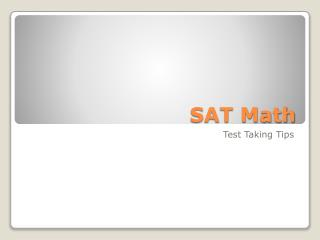Download PresentationSAT Math

# SAT Math

Download Presentation## SAT Math

- - - - - - - - - - - - - - - - - - - - - - - - - - - E N D - - - - - - - - - - - - - - - - - - - - - - - - - - -
##### Presentation Transcript

1. SAT Math Test Taking Tips

2. It’s NOT about the math. Just because you get As in Algebra and Geometry does not ensure you’ll get a great score! • The most difficult part of the math is FINISHING! • Preparation makes a bigger difference in math than verbal.

3. Problems often have complicated directions with unexpected turns. • Some have obvious answers that are wrong. • Others build in a final step – often forgotten. • Unlike the PSSAs, the SATs care NOTHING about the process. They care only about the correct answer.

4. So how do I prepare? What do I do that day?

5. Practice timed tests.

6. Know YOUR calculator!

7. Be familiar with the formulas that are provided.

8. What’s It Look Like

9. Total questions = 54 44 are Multiple Choice 10 are Student Produced Answers (Grid-ins)

10. Each “REAL” SAT contains one 25 minute section used to develop future test questions It is NOT scored. You do NOT know which section.

11. Quick Refresher

12. http://ca.youtube.com/watch?v=uydzT_WiRz4

13. Even + Even • Even + Odd • Odd + Even • Odd + Odd Addition of Integers

14. Even x Even • Even x Odd • Odd x Odd Multiplication of Integers

15. Know the square of every number from 1 to 15! • 1,4, 9,16, 25, 36, 49, 64, 81,100, 121, 144, 169, 196, 225 Squares

16. Know the cubes of every whole number from 1 to 5 • 1,8,27,64,125 CUBES

17. Square root of 1 = 1 • Square root of 2 = 1.4 • Square root of 3 = 1.7 • Square root of 4 = 2 • Square root of 5 = 2.25 Square Roots

18. Fractions squared or cubed are smaller than the original. • Negative numbers squared are positive. • Negative numbers cubed are negative.

19. Read each problem carefully. Determine what information you need and what is extraneous. • If you get stuck on a problem skip it.

20. Multiple Choice

21. Don’t use a calculator on every problem. • Expect easy questions at the beginning of each section and harder towards the end. • MAKE SURE you are on the same number as you are on your answer sheet! • Use the edge of your paper as a ruler. • Draw a picture, sketch or figure.

22. Work backwards. Substitute answers in to equations, or in quantitative comparisons substitute numbers. • Estimate! Use round numbers. • Don’t forget about negative numbers! • Your answer may be a different expression than the choices – write it in another form. • If you can eliminate at least one answer guess. • Make sure the answer is reasonable.

23. Grid - ins

24. Relatively small part of the test • No penalty for wrong answers • Positive numbers • Between zero and what?

25. Practice

26. http://www.csmonitor.com/2007/0712/p13s02-legn.html?page=1

27. http://www.collegeboard.com/student/testing/sat/prep_one/math_review.htmlhttp://www.collegeboard.com/student/testing/sat/prep_one/math_review.html

28. http://cte.jhu.edu/techacademy/web/2000/heal/siteslist.htm

29. http://ca.youtube.com/watch?v=y5tOHx9dZ7o

30. http://www.learningmathcenter.com/LMCinterfaceSAT.html

31. http://www.majortests.com/sat/grid-ins.php

32. Delicious.com/mctoth/sat